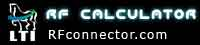#### Symbols Key

A = Ampere

a = Outside radius of center conductor

b = Inside radius of outer conductor

c = Speed of Light

dB = Decibel

dBi = Decibel Isotropic

dBm or dbmW = Decibel to 1 milliwatt

dBmV = Decibel to 1 millivolt

dBW = Decibel to 1 watt

dBV = Decibel to 1 Volt

dBuV = Decibel to 1 microvolt

EIRP = Effective Isotropically Radiated Power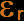= relative permittivity

f= frequency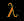= free space wavelength

L = Inductance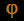= phase length

pT = picoTesla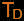= Time Delay

V = Volts

VP = velocity of propagation

VSWR = Voltage Standing Wave Ratio

W = watts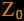= Characteristic Impedance

Please visit RFconnector.com for all of your RF connector and RF cable assembly needs